# Understand Pointer in C++

If you are looking to learn about pointer in C/C++. Here is the right place for you. Understanding the pointer can be confusing at first time, but you will get the hang of it. I will provide some illustrations and examples to get better a picture about a pointer. There are many other pointers available, they are called Smart Pointers, but for the interest of this article I will be discussing the basic Pointer in C++.

What is a Pointer?

As the name suggests, basically something that points at something. This is pretty much what pointer in C++ does.

Let me describe this in a technical term. A pointer is a variable of a type(int, double, boolean, Array, etc) which holds the address of another variable of a same type, and some times the pointer can be pointed to NULL. Not only pointer has access to the address, and also value of the pointed variable as well.

How to create a pointer, and store the address of a variable?

In C++ you can create a pointer variable just like any other variable. You specify the type (int, float, boolean. Etc)you are going to point at, after that you have to add a star (*) symbol then followed by the name of the variable, and then you can set equal to the variable you want to point at. You have to add an ampersand (&) before the variable name to indicate that you are storing the address of a variable id.

An example of a pointer in C/C++.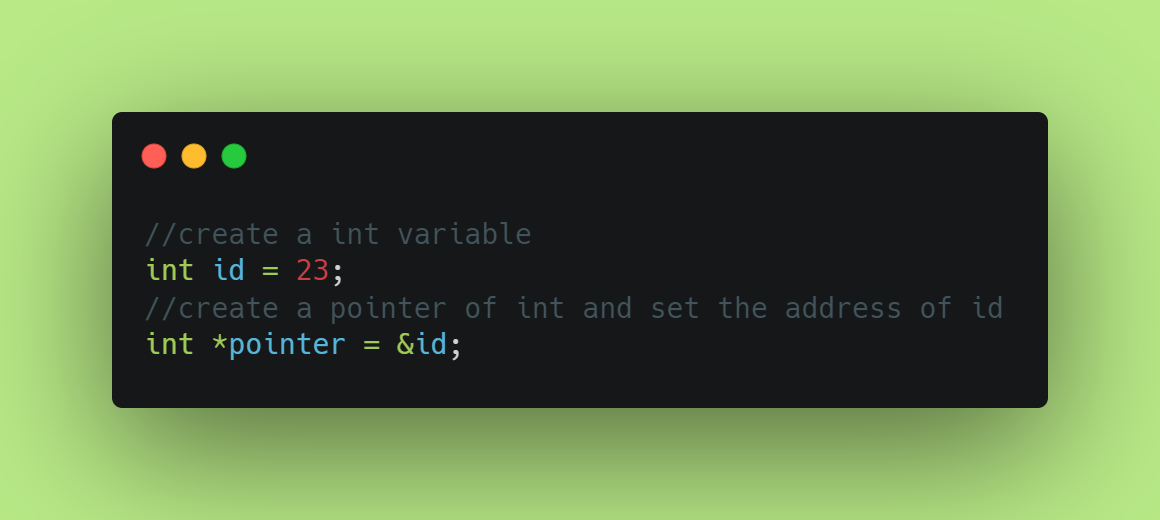Pointer example

As you can see in the image, we have declared id as a type int, and has a value of 23. Then, we have created a pointer type with name of pointer which has a value of address of id.

As you can observer, the pointer variable type, and other variable type is the same. If you have pointer variable type float, you cannot store the address of a variable type of int.

Illustration below might help you to understand about a pointer.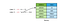Pointer that’s storing the address of variable id.

Pointer variable is holding the address of variable id. If you try to cout (Print the value on the console) the pointer variable, you will see the address of a variable id, not the value of it which is 23. To print the address of a pointer variable, you do not have to add “*” front of the pointer variable name. To see the address of a variable you can write the following code :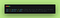Illustration of Pointer.

If you are trying to print the pointer variable, the address of id which is x0A234 in this case will be printed on the screen. The address illustrated above in the memory table is not the same address as you will see in your console.

We know how to get the address of a variable, but how to get the value of the variable? Which is in this case 23.

To get the value of the variable we have to use the dereference operator (*). When you use the dereference operator, we are actually getting the value of the variable not a copy of it. Following illustrates how to use dereference operator to get the value of pointed variable.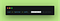Derefrencing the pointer

We have to add (*) operator front before the pointer variable name to get the value of the pointed variable. Since we are getting the actual value, we can also change as well, and it will actually be changing the value of the pointed value. Following code demonstrate this.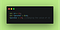Changing the value.

As you can use that we are using dereference operator to change the value of a variable id. If you cout variable id the output will be 24 not 23, its because pointer is pointing at the original value not the copy it, since it has that ability it also has the ability to change the value of it as well.

Summary:

• Pointer stores the address of a variable

I hope this tutorial was helpful. If it was please clap. If you think I have made any mistake, please let me know.

Thank You.

Reference:

http://www.cplusplus.com/doc/tutorial/pointers/

Like to write about stuff.

## More from Mohamed Abdul

Like to write about stuff.